# 5. (5 pts.) The current spot exchange rate is \$1.55 1.00 and the three-month forward rate is \$1.6...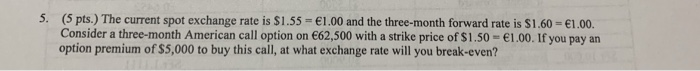5. (5 pts.) The current spot exchange rate is \$1.55 1.00 and the three-month forward rate is \$1.60 1.00. Consider a three-month American call option on €62.500 with a strike price of S 1.50·ei .00, if you pay an option premium of \$5,000 to buy this call, at what exchange rate will you break-even?

Breakeven exchange rate is given as equal to

=5000/62500+1.50=1.58\$/€

##### Add Answer of: 5. (5 pts.) The current spot exchange rate is \$1.55 1.00 and the three-month forward rate is \$1.6...
Similar Homework Help Questions
• ### The current spot exchange rate is \$1.55/€ and the three-month forward rate is \$1.60/€. Consider a...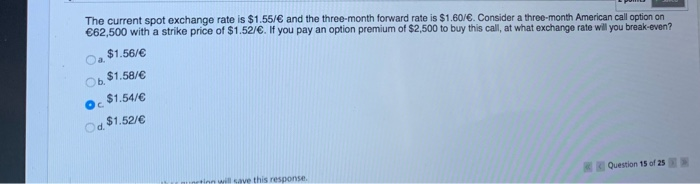The current spot exchange rate is \$1.55/€ and the three-month forward rate is \$1.60/€. Consider a three-month American call option on €62,500 with a strike price of \$1.52/€. If you pay an option premium of \$2,500 to buy this call, at what exchange rate will you break-even? a \$1.56/€ O b. \$1.58/€ o \$1.54/€ Od \$1.521€ Question 15 of 25 S a ve this response

• ### The current spot exchange rate is \$1.55/€ and the three-month forward rate is \$1.50/€. You are...

The current spot exchange rate is \$1.55/€ and the three-month forward rate is \$1.50/€. You are selling €1,000 forward for \$. How much in \$ are you receiving in three months? If the spot exchange rate is \$1.60/€ in three months, how much is the gain or loss from the forward hedge?

• ### The current spot exchange rate is \$1.50/€ and the three-month forward rate is \$1.55/€. Based on...

The current spot exchange rate is \$1.50/€ and the three-month forward rate is \$1.55/€. Based on your analysis of the exchange rate, you are confident that the spot exchange rate will be \$1.62/€ in three months. Assume that you would like to buy or sell €1,000,000. What actions do you need to take to speculate in the forward market? What is the expected dollar profit from speculation? A) Sell €1,000,000 forward for \$1.50/€. B) Buy €1,000,000 forward for \$1.55/€. C)...

• ### 19 Suppose that the GBP is pegged to gold at £20 per ounce. The USD is...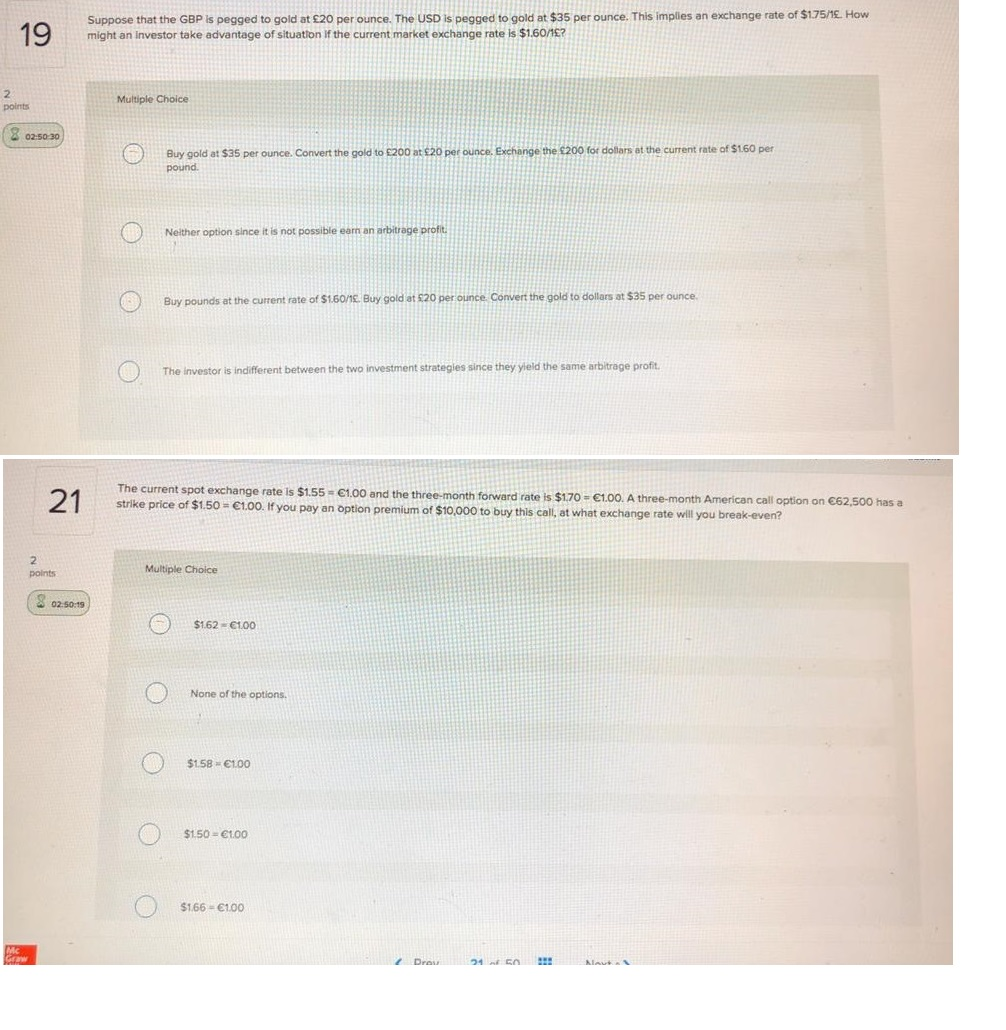19 Suppose that the GBP is pegged to gold at £20 per ounce. The USD is pegged to gold at \$35 per ounce. This implies an exchange rate of \$175/18. How might an Investor take advantage of situation if the current market exchange rate is \$1.60/1£? Multiple Choice points 02-50-30 0 Buy gold at \$35 per ounce. Convert the gold to \$200 at \$20 per ounce. Exchange the €200 for dollars at the current rate of \$160 per pound 0...

• ### 7. The current spot exchange rate is \$1.95/£ and the three-month forward rate is \$1.90/£. Based...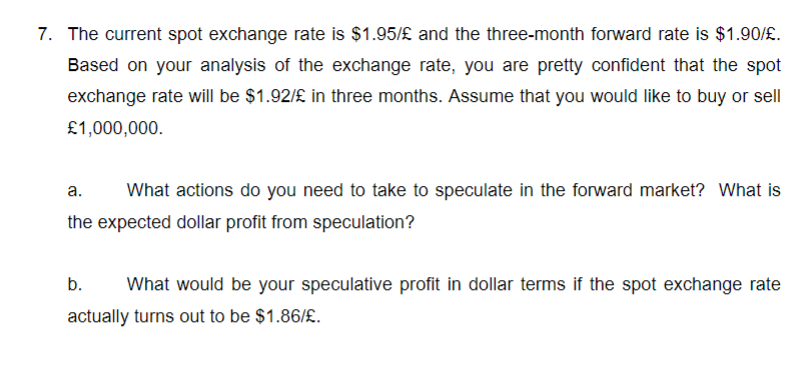7. The current spot exchange rate is \$1.95/£ and the three-month forward rate is \$1.90/£. Based on your analysis of the exchange rate, you are pretty confident that the spot exchange rate will be \$1.92/£ in three months. Assume that you would like to buy or sell £1,000,000 a. What actions do you need to take to speculate in the forward market? What is the expected dollar profit from speculation? b. What would be your speculative profit in dollar terms...

• ### The current spot exchange rate is \$1.45/£. The three-month forward rate is \$1.40/£. An investor shorts...

The current spot exchange rate is \$1.45/£. The three-month forward rate is \$1.40/£. An investor shorts £1,260. At maturity, the spot rate is \$1.50/£. The gain or loss (−) on this transaction is: Multiple Choice \$126. £126. −\$126. None of the options. −£126.

• ### Suppose that the current spot exchange rate is 0.80/\$ and the three-month forward exchange rate i...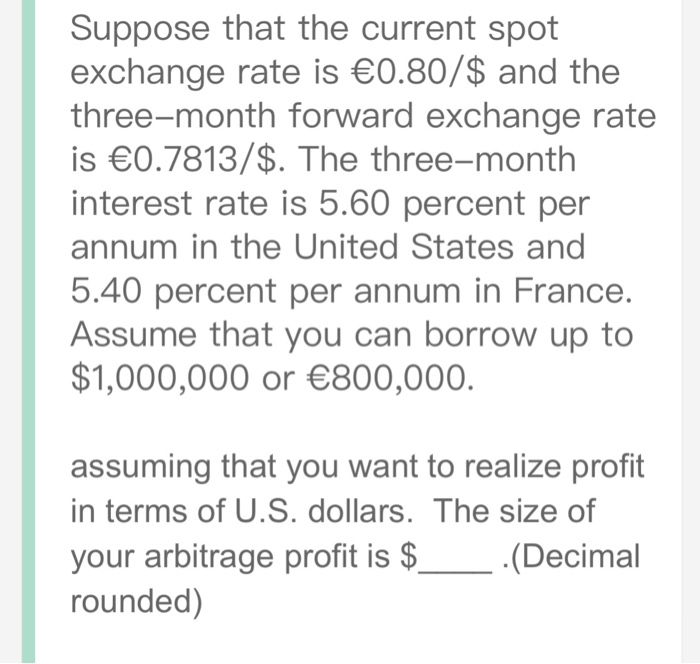Suppose that the current spot exchange rate is 0.80/\$ and the three-month forward exchange rate is 0.7813/\$. The three-month interest rate is 5.60 percent per annum in the United States and 5.40 percent per annum in France. Assume that you can borrow up to \$1,000,000 or 800,000. assuming that you want to realize profit in terms of U.S. dollars. The size of your arbitrage profit is S rounded) Suppose that the current spot exchange rate is 0.80/\$ and the three-month...

• ### Suppose that the current spot exchange rate is €0.8250/\$ and the three month forward exchange rate...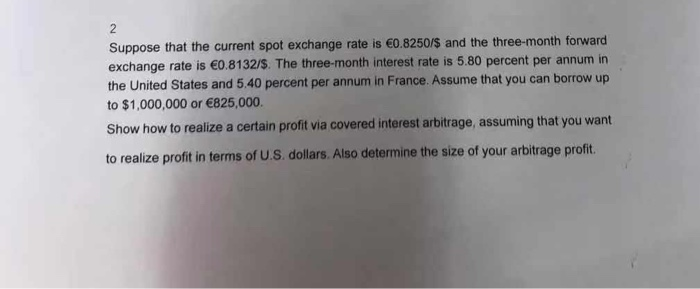Suppose that the current spot exchange rate is €0.8250/\$ and the three month forward exchange rate is €0.8132/\$. The three-month interest rate is 5.80 percent per annum in the United States and 5.40 percent per annum in France. Assume that you can borrow up to \$1,000,000 or €825,000. Show how to realize a certain profit via covered interest arbitrage, assuming that you want to realize profit in terms of U.S. dollars. Also determine the size of your arbitrage profit

• ### Suppose that the current spot exchange rate is €0.8250/\$ and the three month forward exchange rate...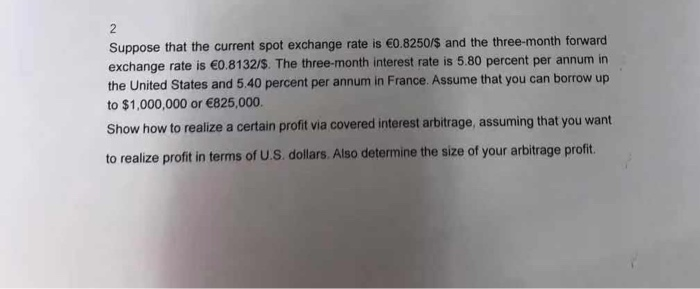Suppose that the current spot exchange rate is €0.8250/\$ and the three month forward exchange rate is €0.8132/\$. The three-month interest rate is 5.80 percent per annum in the United States and 5.40 percent per annum in France. Assume that you can borrow up to \$1,000,000 or €825,000. Show how to realize a certain profit via covered interest arbitrage, assuming that you want to realize profit in terms of U.S. dollars. Also determine the size of your arbitrage profit

• ### 3. Currently, the spot exchange rate is \$1.50/£ and the three-month forward exchange rate is \$1.52/£....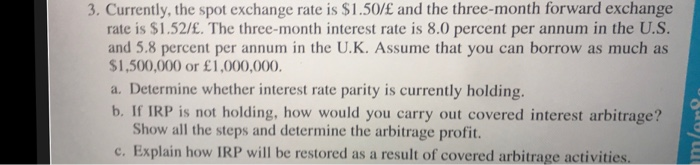3. Currently, the spot exchange rate is \$1.50/£ and the three-month forward exchange rate is \$1.52/£. The three-month interest rate is 8.0 percent per annum in the U.S. and 5.8 percent per annum in the U.K. Assume that you can borrow as much as \$1,500,000 or £1,000,000. a. Determine whether interest rate parity is currently holding. b. If IRP is not holding, how would you carry out covered interest arbitrage? Show all the steps and determine the arbitrage profit. c....

Need Online Homework Help?